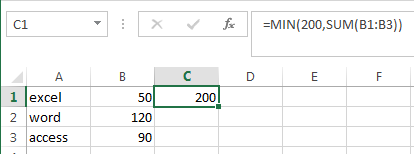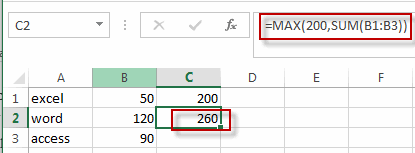# Limit Formula Result to Maximum or Minimum Value

This post will guide you how to limit formula result to a maximum or minimum value in excel. For example, if you want to limit the results of a formula to a maximum value of 200, how to this this in excel. How do I return a formula result only within a specified minimum or maximum values in excel. Do we have any ways to limit the results of a calculation to a maximum value.

You can use the MIN function or Max function to create a formula to limit a number within a minimum or maximum value. Just do the following steps:

## 1. Limit Formula Result to Maximum Value

If you want to limit a formula result to a maximum value 200, you can use the MIN function to create a formula, just like this:

``=MIN(200,SUM(B1:B3))``If the result of SUM function is greater than 200, then the MIN function will return value 200. Otherwise, return the result of SUM function.

## 2. Limit Formula Result to Minimum value

If you want to limit a formula result (for example, the sum function) to a minimum value, then you can use the MAX function in combination with the SUM function or other function to create a new formula. Just like this:

``=MAX(200,SUM(B1:B3))``If the result returned by the SUM function is smaller than 200, then returns value 200, otherwise, it will return the result returned by the SUM function.

## 3. Video: Limit Formula Result to Maximum or Minimum Value in Excel

This video will demonstrate how to limit the result of a formula to a specific minimum or maximum value using the MIN Function or MAX Function in Excel.

## 4. Related Functions

• Excel SUM function
The Excel SUM function will adds all numbers in a range of cells and returns the sum of these values. You can add individual values, cell references or ranges in excel.The syntax of the SUM function is as below:= SUM(number1,[number2],…)…
• Excel MIN function
The Excel MIN function returns the smallest numeric value from the numbers that you provided. Or returns the smallest value in the array.The MIN function is a build-in function in Microsoft Excel and it is categorized as a Statistical Function.The syntax of the MIN function is as below:= MIN(num1,[num2,…numn])….
• Excel MAX function
The Excel MAX function returns the largest numeric value from the numbers that you provided. Or returns the largest value in the array.= MAX(num1,[num2,…numn])…